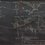# 2 original proofs that harmonic series diverges

1. All positive integers but $$1$$ are divisble by at least one prime. The probability an integer is divisble by $$p$$ is $$\frac{1}{p}$$; the complement of that is $$1-\frac{1}{p}$$ and so the product over all primes $$\displaystyle \prod_{p \ \text{prime}} \left( 1-\frac{1}{p} \right) = 0$$. From the Euler product, we thus know $$\zeta(1)$$ is divergent.

2. Assume the harmonic series converges; then it converges absolutely, since all its terms are positive. This is obviously a contradiction, since we can tell it is conditionally convergent from the ratio/root test.Note by Jake Lai
5 years, 11 months ago

This discussion board is a place to discuss our Daily Challenges and the math and science related to those challenges. Explanations are more than just a solution — they should explain the steps and thinking strategies that you used to obtain the solution. Comments should further the discussion of math and science.

When posting on Brilliant:

• Use the emojis to react to an explanation, whether you're congratulating a job well done , or just really confused .
• Ask specific questions about the challenge or the steps in somebody's explanation. Well-posed questions can add a lot to the discussion, but posting "I don't understand!" doesn't help anyone.
• Try to contribute something new to the discussion, whether it is an extension, generalization or other idea related to the challenge.
• Stay on topic — we're all here to learn more about math and science, not to hear about your favorite get-rich-quick scheme or current world events.

MarkdownAppears as
*italics* or _italics_ italics
**bold** or __bold__ bold
- bulleted- list
• bulleted
• list
1. numbered2. list
1. numbered
2. list
Note: you must add a full line of space before and after lists for them to show up correctly
paragraph 1paragraph 2

paragraph 1

paragraph 2

[example link](https://brilliant.org)example link
> This is a quote
This is a quote
    # I indented these lines
# 4 spaces, and now they show
# up as a code block.

print "hello world"
# I indented these lines
# 4 spaces, and now they show
# up as a code block.

print "hello world"
MathAppears as
Remember to wrap math in $$ ... $$ or $ ... $ to ensure proper formatting.
2 \times 3 $2 \times 3$
2^{34} $2^{34}$
a_{i-1} $a_{i-1}$
\frac{2}{3} $\frac{2}{3}$
\sqrt{2} $\sqrt{2}$
\sum_{i=1}^3 $\sum_{i=1}^3$
\sin \theta $\sin \theta$
\boxed{123} $\boxed{123}$

## Comments

Sort by:

Top Newest

Your proof #1 just went over my head since I'm not quite familiar with Euler product and stuff yet. So, I won't comment on that.

Regarding your proof #2, how can you "tell it is conditionally convergent from the ratio/root test" ? Both ratio and root tests can only be used to test either absolute convergence or divergence. In other cases when the criteria for divergence or absolute convergence is not met, the test is inconclusive. When the tests are inconclusive, the sum can be convergent (absolute / conditional) or divergent. You cannot say for sure that the series must be conditionally convergent using ratio / root test in such inconclusive cases (as far as I know). I'm saying all this based on the Wikipedia articles on ratio / root tests.

Correct me if I'm wrong.

- 5 years, 11 months ago

Log in to reply

My proof for divergence requires no calculus.

$\dfrac {1}{1} + \dfrac {1}{2} + \dfrac {1}{3} + \dfrac {1}{4} + \dfrac {1}{5} + \dfrac {1}{6} + \dfrac {1}{7} + \dfrac {1}{8} + \ldots > \dfrac {1}{1} + \dfrac {1}{2} + \dfrac {1}{4} + \dfrac {1}{4} + \dfrac {1}{8} + \dfrac {1}{8} + \dfrac {1}{8} + \dfrac {1}{8} + \ldots$

$\dfrac {1}{1} + \dfrac {1}{2} + \dfrac {1}{3} + \dfrac {1}{4} + \dfrac {1}{5} + \dfrac {1}{6} + \dfrac {1}{7} + \dfrac {1}{8} + \ldots > \dfrac {1}{1} + \dfrac {1}{2} + \left( \dfrac {1}{4} + \dfrac {1}{4} \right) + \left(\dfrac {1}{8} + \dfrac {1}{8} + \dfrac {1}{8} + \dfrac {1}{8} \right) + \ldots$

$\dfrac {1}{1} + \dfrac {1}{2} + \dfrac {1}{3} + \dfrac {1}{4} + \dfrac {1}{5} + \dfrac {1}{6} + \dfrac {1}{7} + \dfrac {1}{8} + \ldots > \dfrac {1}{1} + \dfrac {1}{2} + \dfrac {1}{2} + \dfrac {1}{2} + \ldots$

$\dfrac {1}{1} + \dfrac {1}{2} + \dfrac {1}{3} + \dfrac {1}{4} + \dfrac {1}{5} + \dfrac {1}{6} + \dfrac {1}{7} + \dfrac {1}{8} + \ldots > \infty$

Hence proven.

- 5 years, 11 months ago

Log in to reply

Right! This is the classical proof of divergence of harmonic series using comparison. This proof is also featured in the Wikipedia page on Harmonic series. Hence, not an original proof.

- 5 years, 11 months ago

Log in to reply

Ye, but nonetheless, easy to remember.

- 5 years, 11 months ago

Log in to reply

Oresme reincarnate!

- 5 years, 11 months ago

Log in to reply

I'm surprised I know his name. Wasn't Nicole Oresme an astronomer, a mathematician and physicist?

- 5 years, 11 months ago

Log in to reply

Your proof is often attributed to Oresme.

- 5 years, 11 months ago

Log in to reply

Oh

- 5 years, 11 months ago

Log in to reply

×

Problem Loading...

Note Loading...

Set Loading...